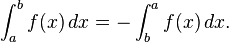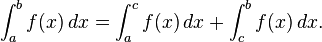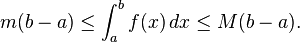## Properties of integrals

1.  the integral of a linear combination is the linear combination of the integrals,If a > b then define3.

Additivity of integration on intervals. If c is any element of [a, b], then4.
Upper and lower bounds. An integrable function f on [a, b], is necessarily bounded on that interval. Thus there are real numbers m and M so that mf (x) ≤ M for all x in [a, b]. Since the lower and upper sums of f over [a, b] are therefore bounded by, respectively, m(ba) and
M(ba), it follows thatApplications in physics/technology of integrals.

Annonser## Om mattelararen

Licentiate of Philosophy in atomic Physics Master of Science in Physics
Det här inlägget postades i Calculus, matematik 3c, matematik 4 och har märkts med etiketterna . Bokmärk permalänken.